# Frac equation v2

3.80 is two fifths of a quarter sum of money. How much money is there?

x =  39

### Step-by-step explanation: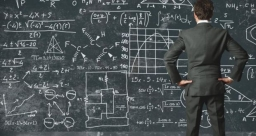Did you find an error or inaccuracy? Feel free to write us. Thank you!Tips to related online calculators
Need help to calculate sum, simplify or multiply fractions? Try our fraction calculator.
Do you have a linear equation or system of equations and looking for its solution? Or do you have a quadratic equation?

## Related math problems and questions:

• What is 9What is the value of x in the proportion 2 and one-fourth over x = 1 and one-half over 3 and three-fifths? 2 and two-fifths 5 and two-fifths 8 and 1 over 10 12 and 3 over 20
• Eq-fracSolve the following equation with fractions: h + 1/3 =5/3
• TreesFrom the total number of trees in the orchard, there are two-fifths pearls and apples are three eighty. The rest of the trees are 9 ceremonial. How many trees are in the set?
• EvaluateThe division of numbers $a and$b increase by-product of the numbers $c and$d
• The sumThe sum of five consecutive odd numbers is 75. Find out the sum of the second and fourth of them.
• Two-fifths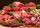Two-fifths (2/5) of a pound of chicken for each person. If there are 8 people to be fed, how many pounds of chicken are required?
• Pupils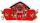Two classes want to collect money. Boys are four-seventh pupils. In time did not pay a quarter of the boys and a sixth of girls, which mean 12 sinners. How many pupils attend these two classes?
• The vase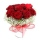There were roses in the vase, half were red, a quarter was white and 12 were yellow. How many roses were in the vase?
• Sum of 18Sum of two fractions is 4 3/7. If one of the fractions is 2 1/5 find the other one .
• Shop stores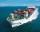Workers untied fruit to stores. In the first quarter shipments, in the second fifth shipment, in the third two-fifths of the rest and in the fourth 231 kilograms. How many fruits were together?
• Two numbers 11The sum of two rational numbers is (-2). If one of them is 3/5, find the other.
• PearsThere were pears in the basket, I took two-fifths of them, and left six in the basket. How many pears did I take?
• Two numbersThe sum of two numbers is 1. Identify this two numbers if you know that the half of first is equal to the third of second number.
• Quarter circularThe wire that is hooked around the perimeter of quarter-circular arc has length 3π+12. Determine the radius of circle arc.
• Worker salaryThe worker had a salary of CZK 18,000. During the year, his salary was increased by a quarter. He earned a total of CZK 247,500 for the whole year. From which month was his salary increased?
• Four friendsFour friends shared the money. Vasek got 1/4 of the total amount. Tonda received 1/3 of the rest of the money, Joe got a half from the second residue and Jirka left 80. How much money get together?
• Trees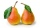Two-fifths of the trees in the orchard are apple trees, one-sixth a pear. The remaining 26 trees are cherries. How many pears are in the orchard?